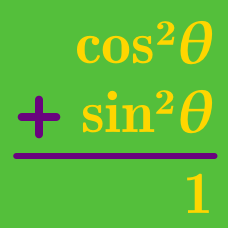Geometry

# Basic Proving Identities using Pythagorean Theorem

Which of the following is equal to $1-\cos^2\theta?$

Which of the following is equal to $1-\sin^2\theta?$

Which of the following is equal to $(1-\cos\theta)(1+\cos\theta)?$

Which of the following is equal to $(\sin\theta-1)(\sin\theta+1)?$

Which of the following is equal to $\dfrac{\sec^2\theta}{\tan^2\theta+1}?$

×